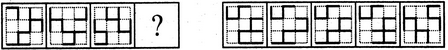# Non Verbal Reasoning - Analogy - Discussion

### Discussion :: Analogy - Section 2 (Q.No.35)

Each of the following questions consists of two sets of figures. Figures A, B, C and D constitute the Problem Set while figures 1, 2, 3, 4 and 5 constitute the Answer Set. There is a definite relationship between figures A and B. Establish a similar relationship between figures C and D by selecting a suitable figure from the Answer Set that would replace the question mark (?) in fig. (D).

35.

Select a suitable figure from the Answer Figures that would replace the question mark (?).(A)     (B)      (C)     (D)                  (1)      (2)      (3)      (4)      (5)

 [A]. 1 [B]. 2 [C]. 3 [D]. 4 [E]. 5

Explanation:

The figure rotates 90oCW.

 Muneer said: (Aug 25, 2019) Can't understand it. Explain it in detail.